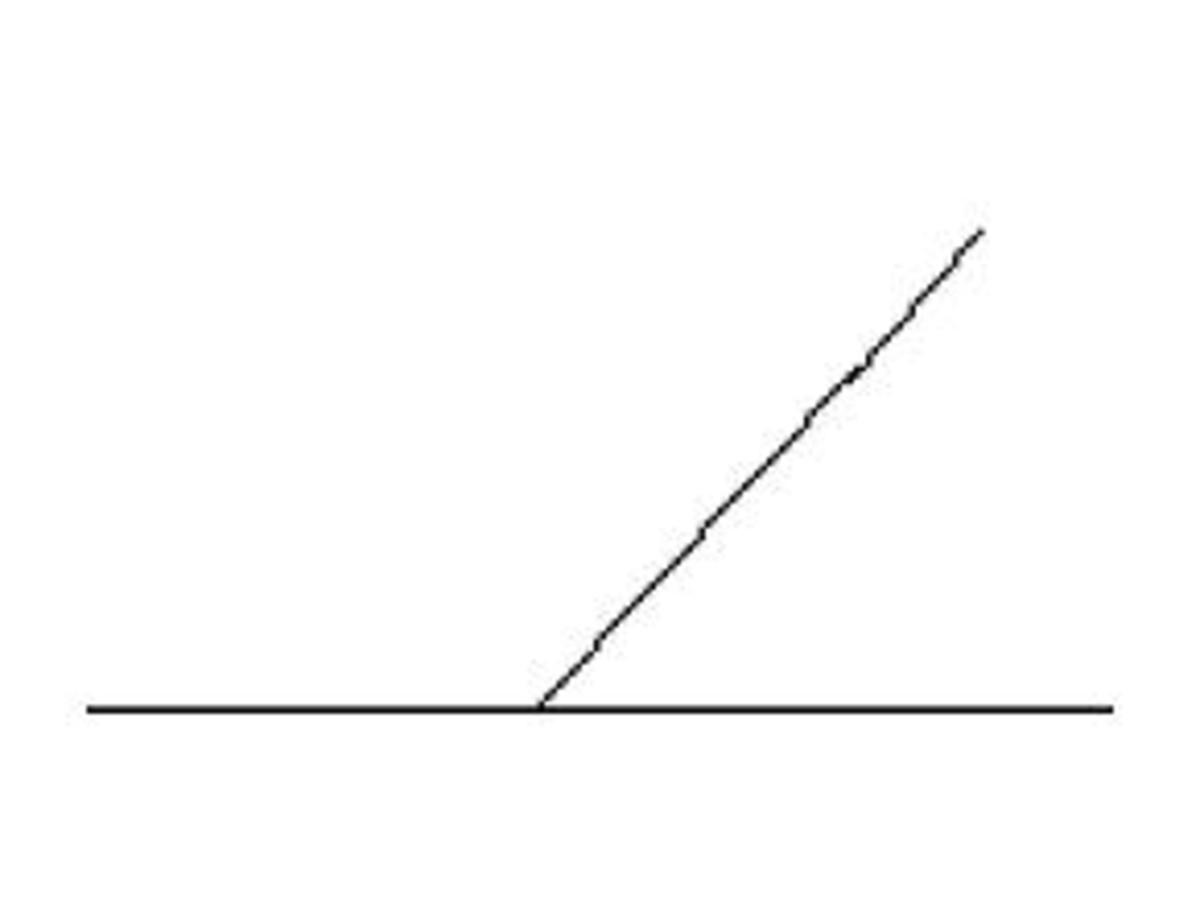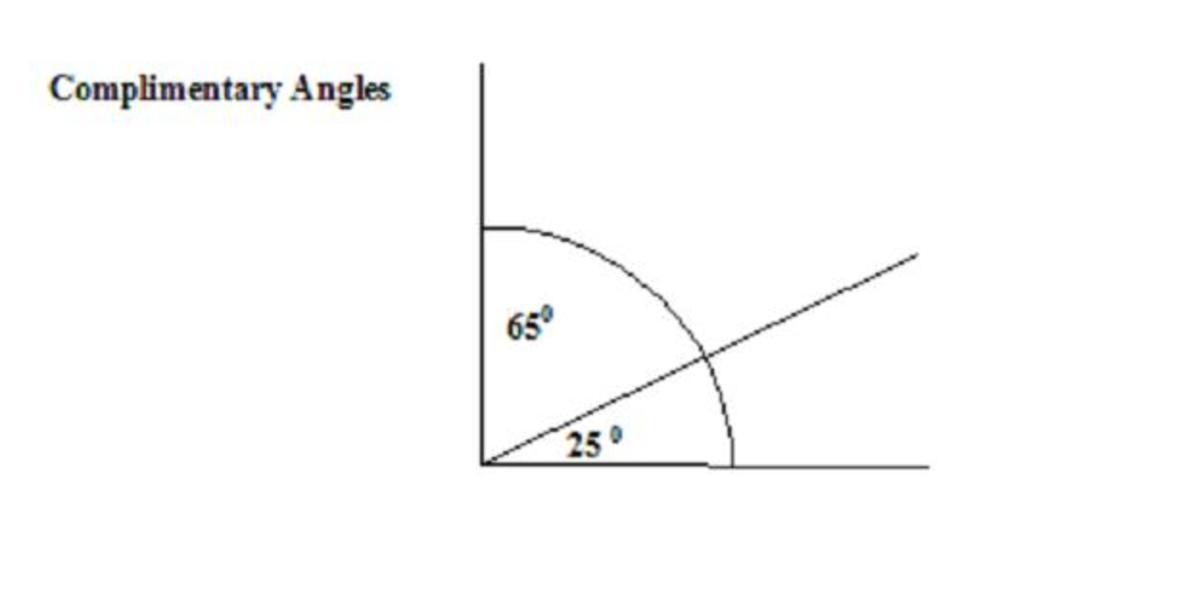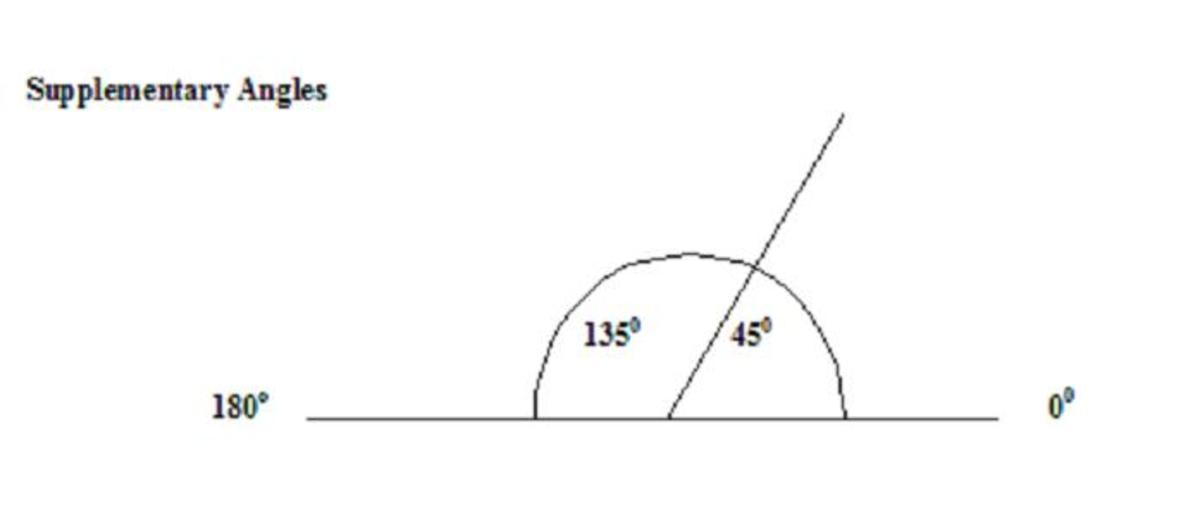# How to Draw and Measure AnglesStart off by drawing a baseline with ruler and pencil, then draw a second line at an angle.Joan WhetzelTwo acute angles equaling 90 degrees are complimentary angles. Here angle BAC = 65 degrees and angle CAD = 25 degrees.Joan WhetzelAn acute and an obtuse angle equaling 180 degrees are supplementary angles. Here angle BAC = 135 degrees and angle CAD = 45 degrees.Joan Whetzel

## By Joan Whetzel

Angle sizes are measured in degrees, as compared to degrees along the circumference of a circle. A full circle measures 360 degrees, a line bisecting the circle along the diameter measures 180 degrees, and an angle that cuts our 1/4 of the circle measures 90 degrees. To understand angles fully, learn how to draw them and how to measure them.

Items Needed

Protractor

Paper

Pencil

Ruler

Types of Angles

Angles are classified according to their proportions. For instance acute angles measure less than 90 degrees while obtuse angles measure greater than 90 but less than 180 degrees. A right angle equals 1/4 of a circle or 90 degrees, with the lines of the angle running perpendicular to each other. Supplementary angles occur when an acute and an obtuse angle are added together to equal 180 degrees. Two acute angles added together to create a 90 degree angles are complimentary angles.

Drawing an angle

To draw an angle, use the pencil and ruler to draw a horizontal baseline on a piece of paper. This creates a 180 degree angle. Mark the center of the baseline. Use the pencil and ruler to draw another line at an angle beginning from the center of the baseline, and angled upward away from the baseline. This angle forms a supplementary angle or an obtuse/acute angle combination.

Next, draw another horizontal baseline. This time, draw a vertical line at one end of the base line, forming one half of a square, or a right angle. Next use the, use the pencil and ruler to draw another angled line that bisects the right angle. This bisecting line forms a complimentary angle formed by two acute angles.

Scroll to Continue

Labeling the Angle Points

Label each angle point with a capital letter. Mark the point where all the angles converge as "A" and the point at the end of each line with the letters "B", "C", and "D". On the supplementary angle, the end point to the left of "A" is "B". and the endpoints to the right of "A" are "C" and "D." For the Complimentary Angle, the endpoint directly over "A" is endpoint "B" and the other two endpoints become "C" and "D."

These labeled points will be used to identify angle BAC, BD, and CAD.

Using a Protractor

Place the protractor along the baseline, with the center hole lined up with point A. Line the ruler up along Line angle AC to find the degrees along the outer ring. Two numbers will be listed that will equal 180 degrees. The lower number measures the acute angle (angle CAD) and the large number measures the obtuse angle (angle BAC).

To find both acute angles for the right angle, place the center hole on point A of the right angle. Line the ruler up with the angled line to find the degree marker along the outer ring of the protractor. The angle marker measures the size of the smaller angle in degrees, for instance it may read 25 degrees. Subtract 25 degrees from 90 degrees to find the measurement for the other acute angle. Example 900 - 250 = 650. Angle BAC equals 65 degrees and angle CAD measures 25 degrees.

Double check your math by adding the 2 acute angles which should equal 90 degrees (complimentary angle) and adding the acute/obtuse angles to find 180 degrees (supplementary angles).

The ability to measure angles is important to many occupations, namely architecture and engineering. Learning to draw them and measure them accurately includes double checking your math.

## math and angle drawing from Amazon

Jennifer Kirby on March 06, 2012:

Excellent! I could have used this before helping in my 5th grader's class during math time! Voted up, up, up!

Geoff Morova from South Dakota on October 19, 2011:

Very useful. Wish I had it when I was in school!

Ruchira from United States on October 18, 2011:

Thank you for bringing back my school days. It sure was fun making all sorts of angles :)

voted up!